JEE  >  Test: MCQs (One or More Correct Option): Simple Harmonic Motion (Oscillations) | JEE Advanced

# Test: MCQs (One or More Correct Option): Simple Harmonic Motion (Oscillations) | JEE Advanced

Test Description

## 11 Questions MCQ Test Physics 35 Years JEE Main & Advanced Past year Papers | Test: MCQs (One or More Correct Option): Simple Harmonic Motion (Oscillations) | JEE Advanced

Test: MCQs (One or More Correct Option): Simple Harmonic Motion (Oscillations) | JEE Advanced for JEE 2023 is part of Physics 35 Years JEE Main & Advanced Past year Papers preparation. The Test: MCQs (One or More Correct Option): Simple Harmonic Motion (Oscillations) | JEE Advanced questions and answers have been prepared according to the JEE exam syllabus.The Test: MCQs (One or More Correct Option): Simple Harmonic Motion (Oscillations) | JEE Advanced MCQs are made for JEE 2023 Exam. Find important definitions, questions, notes, meanings, examples, exercises, MCQs and online tests for Test: MCQs (One or More Correct Option): Simple Harmonic Motion (Oscillations) | JEE Advanced below.
 1 Crore+ students have signed up on EduRev. Have you?
*Multiple options can be correct
Test: MCQs (One or More Correct Option): Simple Harmonic Motion (Oscillations) | JEE Advanced - Question 1

### A particle executes simple harmonic motion with a frequency. f. The frequency with which its kinetic energy oscillates is

Detailed Solution for Test: MCQs (One or More Correct Option): Simple Harmonic Motion (Oscillations) | JEE Advanced - Question 1

NOTE : During one complete oscillation, the kinetic energy will become maximum twice.
Therefore the frequency of kinetic energy will be 2f.

*Multiple options can be correct
Test: MCQs (One or More Correct Option): Simple Harmonic Motion (Oscillations) | JEE Advanced - Question 2

### A linear harmonic oscillator of force constant 2 × 106 N/m and amplitude 0.01 m has a total mechanical energy of 160 J. Its

Detailed Solution for Test: MCQs (One or More Correct Option): Simple Harmonic Motion (Oscillations) | JEE Advanced - Question 2

The total energy of the oscillator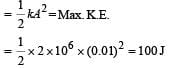As total mechanical energy = 160 J
The P.E. at equilibrium position is not zero.
P.E. at mean position  = (160 – 100) J = 60 J
∴ Max P.E. = (100 + 60 ) J = 160 J.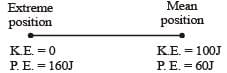*Multiple options can be correct
Test: MCQs (One or More Correct Option): Simple Harmonic Motion (Oscillations) | JEE Advanced - Question 3

### A uniform cylinder of length L and mass M having cross sectional area A is suspended, with its length vertical, from a fixed point by a massless spring, such that it is half- submerged in a liquid of density ρ at equilibrium position. When the cylinder is given a small downward push and released it starts oscillating vertically with small amplitude. If the force constant of the spring is k, the frequency of oscillation of the cylinder is

Detailed Solution for Test: MCQs (One or More Correct Option): Simple Harmonic Motion (Oscillations) | JEE Advanced - Question 3

If x is the displacement then,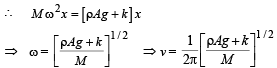*Multiple options can be correct
Test: MCQs (One or More Correct Option): Simple Harmonic Motion (Oscillations) | JEE Advanced - Question 4

A highly rigid cubical block A of small mass M and side L is fixed rigidly on to another cubical block B of the same dimensions and of low modulus of rigidity η such that the lower face of A completely covers the upper face of B. The lower face of B is rigidly held on a horizontal surface. A small force F is applied perpendicular to one of the sides faces of A. After the force is withdrawn, block A executes small oscillations the time period of which is given by

Detailed Solution for Test: MCQs (One or More Correct Option): Simple Harmonic Motion (Oscillations) | JEE Advanced - Question 4

NOTE : When a force is applied on cubical block A in the horizontal direction then the lower block B will get distorted as shown by dotted lines and A will attain a new position (without distortion as A is a rigid body) as shown by dotted lines.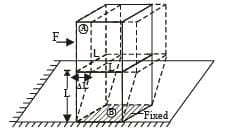For cubical block B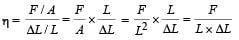⇒ F = ηLΔL

ηL is a constant

⇒ Force F ∝ ΔL and directed towards the mean position, oscillation will be simple harmonic in nature.
Here, Mω2 = ηL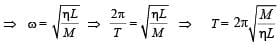*Multiple options can be correct
Test: MCQs (One or More Correct Option): Simple Harmonic Motion (Oscillations) | JEE Advanced - Question 5

One end of a long metallic wire of length L is tied to the ceiling. The other end is tied to a massless spring of spring constant K.A mass m hangs freely from the free end of the spring. The area of cross-section and the Young’s modulus of  the wire are A and Y respectively. If the mass is slightly pulled down and released, it will oscillate with a time period T equal to:

Detailed Solution for Test: MCQs (One or More Correct Option): Simple Harmonic Motion (Oscillations) | JEE Advanced - Question 5

Let us consider the wire also as a spring. Then the case becomes that of two spring attached in series. The equivalent spring constant is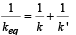where k' is the spring constant of the wire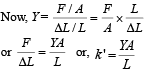We know that time period of the system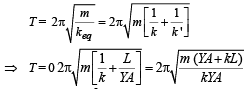*Multiple options can be correct
Test: MCQs (One or More Correct Option): Simple Harmonic Motion (Oscillations) | JEE Advanced - Question 6

A particle of mass m is executing oscillations about the origin on the x axis. Its potential energy is V(x) = k | x |3 where k is a positive constant. If the amplitude of oscillation is a, then its time period  T is

Detailed Solution for Test: MCQs (One or More Correct Option): Simple Harmonic Motion (Oscillations) | JEE Advanced - Question 6

U (x) = k | x |3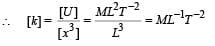Now time period may depend on T ∝ (mass)x (amplitude)y (k)z
∴ [M0L0T] = [M]x [L]y [ML–1 T–2]z
= [Mx + z Ly – z T – 2z]
Equating the powers, we get
– 2z = 1 or  z = – 1/2
y – z = 0  or  y = z = – 1/2
Hence T ∝ (amplitude) –1/2 ∝ a–1/2

*Multiple options can be correct
Test: MCQs (One or More Correct Option): Simple Harmonic Motion (Oscillations) | JEE Advanced - Question 7

Three simple harmonic motions in the same direction having the same amplitude a and same period are superposed. If each differs in phase from the next by 45°, then.

Detailed Solution for Test: MCQs (One or More Correct Option): Simple Harmonic Motion (Oscillations) | JEE Advanced - Question 7

From superposition principle

y = y1 + y2 + y3

= a sin ωt + a sin (ωt + 45°)  + a sin (ωt + 90°)
= a[sin ωt + sin (ωt + 90°] + a sin (ωt + 45°)
= 2a sin (ωt + 45°) cos 45° + a sin (ωt + 45°)

= (√2 +1) a sin (ωt + 45°)
= A sin (ωt + 45°)

Therefore resultant motion is simple harmonic of amplitude A = (√2 +1) a

and which differ in phase by 45° relative to the first.
Energy in SHM ∝ (amplitude)2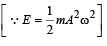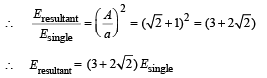*Multiple options can be correct
Test: MCQs (One or More Correct Option): Simple Harmonic Motion (Oscillations) | JEE Advanced - Question 8

The function x = A sin2 ωt + B cos2 ωt + C sin ωt cosωt represent SHM for which of the option(s)

Detailed Solution for Test: MCQs (One or More Correct Option): Simple Harmonic Motion (Oscillations) | JEE Advanced - Question 8

The  given equation is

x = A sin2 ωt + B cos2 ωt + C sin ωt cos ωt

NOTE THIS STEP

Rearranging the equation in a meaningful form (for interpretation of SHM)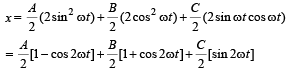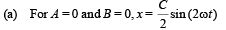The above equation is that of SHM with amplitude C/2 and angular frequency 2ω. Thus option (a) is correct.

(b) If A = B and C = 2B then x = B + B sin 2ωt
This is equation of SHM. The mean position of the particle executing SHM is not at the origin.
Option (b) is correct.
(c) A = – B, C = 2B; Therefore
x = B cos 2ωt + B sin 2ωt
Let B = X cos φ = X sin φ then
x = X sin 2ωt cos φ + X cos 2ωt sin φ This represents equation of SHM.
(d) A = B, C = 0 and x = A.
This equation does not represents SHM.

*Multiple options can be correct
Test: MCQs (One or More Correct Option): Simple Harmonic Motion (Oscillations) | JEE Advanced - Question 9

A metal rod of length ‘L’ and mass ‘m’ is pivoted at one end.
A thin disc of mass ‘M’ and radius ‘R’ (<L) is attached at its center to the free end of the rod. Consider two ways the disc is attached: (case A). The disc is not free to rotate about its centre and (case B) the disc is free to rotate about its centre.
The rod disc system performs SHM in vertical plane after being released from the same displaced position. Which of the following statement(s) is (are) true?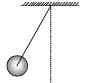Detailed Solution for Test: MCQs (One or More Correct Option): Simple Harmonic Motion (Oscillations) | JEE Advanced - Question 9

Applying τ = Iα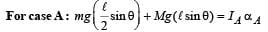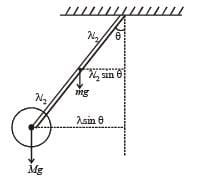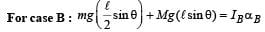The restoring torque in both the cases is same.
Also IA > IB ∴ αA < αB

∴ ωA < wB

(a) and (d) are correct options.

*Multiple options can be correct
Test: MCQs (One or More Correct Option): Simple Harmonic Motion (Oscillations) | JEE Advanced - Question 10

Two independent harmonic oscillators of equal mass are oscillating about the origin with angular frequencies ω1 and ω2 and have total energies E1 and E2, respectively. The variations of their momenta p with positions x are shown in the figures.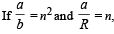then the correct equation(s) is(are)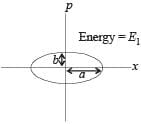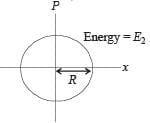Detailed Solution for Test: MCQs (One or More Correct Option): Simple Harmonic Motion (Oscillations) | JEE Advanced - Question 10

Maximum linear momentum in case 1 is (p1)max = mvmax
b = m [aw1] ...(i)
Maximum linear momentum in case 2 is (p2)max = mvmax
R = m [Rω2]

∴ 1 = mω2     ...(ii)

Dividing (i) & (ii)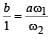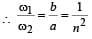∴ B is a correct option.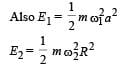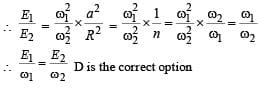*Multiple options can be correct
Test: MCQs (One or More Correct Option): Simple Harmonic Motion (Oscillations) | JEE Advanced - Question 11

A block with mass M is conn ected by a massless spring with stiffness constant k to a rigid wall and moves without friction on a horizontal surface. The block oscillates with small amplitude A about an equilibrium position x0. Consider two cases: (i) when the block is at x0; and (ii) when the block is at x = x0 + A. In both the cases, a particle with mass m(<M) is  softly placed on the block after which they stick to each other. Which of the following statement(s) is (are) true about the motion after the mass m is placed on the mass M?

Detailed Solution for Test: MCQs (One or More Correct Option): Simple Harmonic Motion (Oscillations) | JEE Advanced - Question 11

Case (i) : Applying conservation of linear momentum.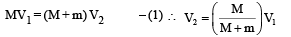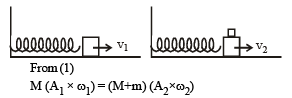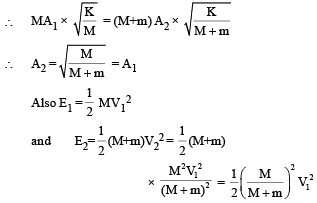Clearly E2 < E1
The new time Period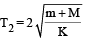Case (ii) : The new time Period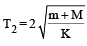Also A2 = A1
Here E2 = E1
The instantaneous value of speed at Xo of the combined masses decreases in both the cases.

## Physics 35 Years JEE Main & Advanced Past year Papers

110 docs|49 tests
 Use Code STAYHOME200 and get INR 200 additional OFF Use Coupon Code
Information about Test: MCQs (One or More Correct Option): Simple Harmonic Motion (Oscillations) | JEE Advanced Page
In this test you can find the Exam questions for Test: MCQs (One or More Correct Option): Simple Harmonic Motion (Oscillations) | JEE Advanced solved & explained in the simplest way possible. Besides giving Questions and answers for Test: MCQs (One or More Correct Option): Simple Harmonic Motion (Oscillations) | JEE Advanced, EduRev gives you an ample number of Online tests for practice

## Physics 35 Years JEE Main & Advanced Past year Papers

110 docs|49 tests